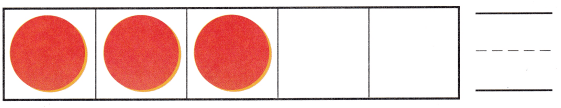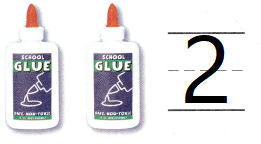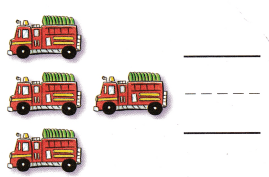# Texas Go Math Kindergarten Module 1 Assessment Answer Key

Refer to our Texas Go Math Kindergarten Answer Key Pdf to score good marks in the exams. Test yourself by practicing the problems from Texas Go Math Kindergarten Module 1 Assessment Answer Key.

## Texas Go Math Kindergarten Module 1 Assessment Answer Key

Concepts and Skills

DIRECTIONS: 1-4. Count how many. Write the number. (TEKS K.2.B)

Question 1.Explanation:
Counted the circles and traced the number

Question 2.Explanation:
Counted the circles and traced the number

Question 3.Explanation:
Counted the Bottles and traced the number

Question 4.Explanation:
Counted the trucks and traced the number

DIRECTIONS: 5. Three children each bring one book to school. Draw counters to show the books Write the number. (TEKS K.2.G) 6. Choose the answer. Which number shows how many books? (TEKS K.2.B)

Question 5.Explanation:
Three children each bring one book to school.
Drawn counters to show the books Written the number as 3

Question 6.
Texas Test Prep